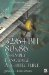# Function yMIN(p, q) Minimum## Function y=MIN(p, q) 'Minimum'

In C code you may find a macro definition similar to:

` #define MIN(p, q) ((p) < (q) ? (p) : (q)) `

But it can be easily replaced in C without the condition test:

` __inline MIN(int p, int q) {     int r;         // r=(p < q) ? p : q     r = (pq) >> INT_MAX_BITS;      // ()=0xFFFFFFFF                                     // (+)=0x00000000     return (p & r)  (q & (r^1));  // keep lower of p or q } `

Normally there would be a single branch test, but as you will note in the C example above and in the assembly below, there is no branch.

``` MIN PROC C PUBLIC p:DWORD, q:DWORD     mov edx,p               ; edx = p     mov ecx,q               ; ecx = q             ; r=(p < q) ? p : q         ; r = (pq) >> INT_MAX_BITS;         ; ()=0xFFFFFFFF (+)=0x00000000     mov eax,edx     sub eax,ecx     sar eax,INT_MAX_BITS    ; (int >> 31)             ; return (p   r)   (q   (

```32/64-Bit 80x86 Assembly Language Architecture
ISBN: 1598220020
EAN: 2147483647
Year: 2003
Pages: 191

Similar book on Amazon